Скачать презентацию Chapter 10 The Cost of Capital Sources

4c39a4026fc0145a71828ea2f4ee8cb1.ppt

• Количество слайдов: 27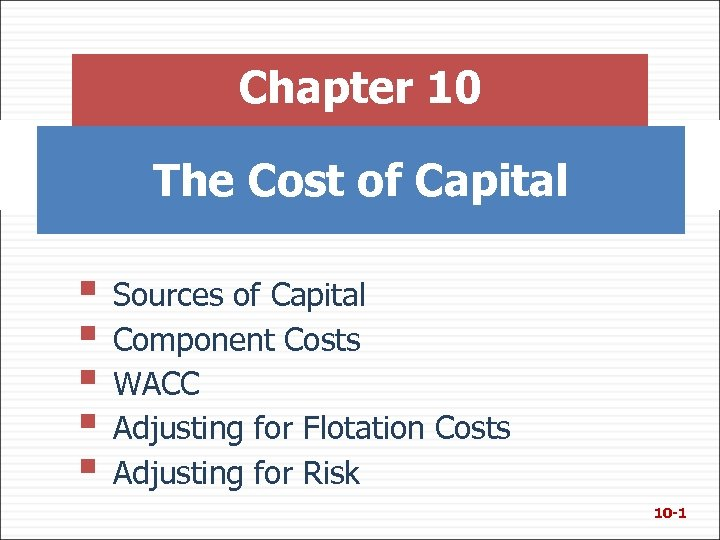Chapter 10 The Cost of Capital § Sources of Capital § Component Costs § WACC § Adjusting for Flotation Costs § Adjusting for Risk 10 -1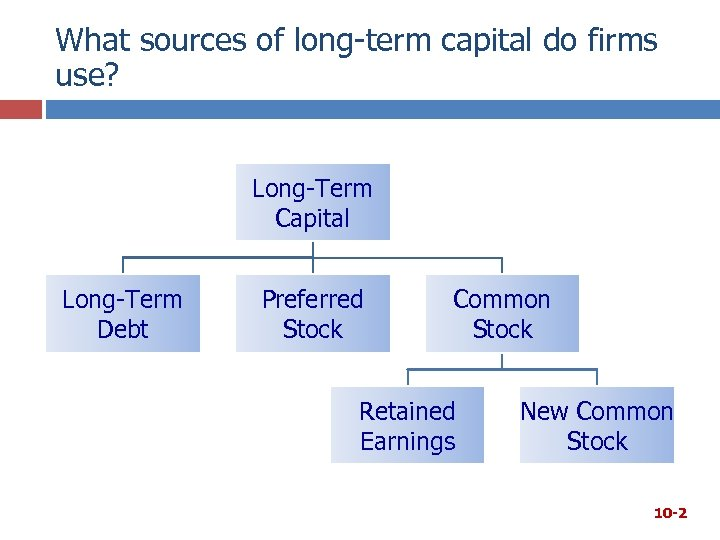What sources of long-term capital do firms use? Long-Term Capital Long-Term Debt Preferred Stock Common Stock Retained Earnings New Common Stock 10 -2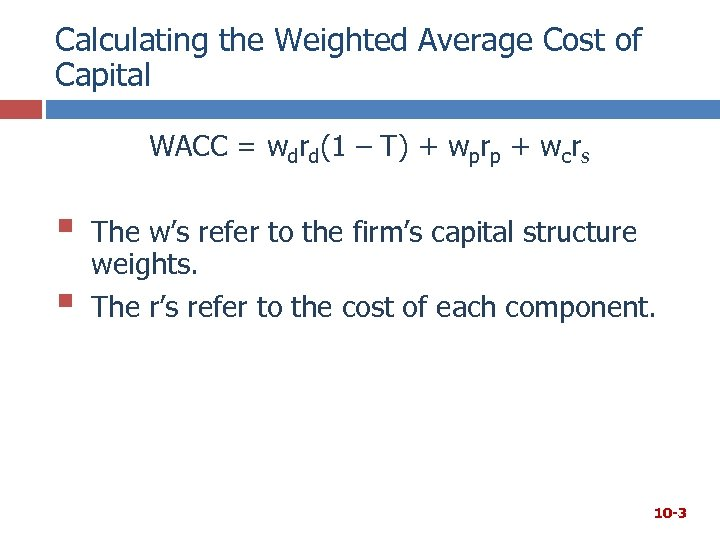Calculating the Weighted Average Cost of Capital WACC = wdrd(1 – T) + wprp + wcrs § § The w’s refer to the firm’s capital structure weights. The r’s refer to the cost of each component. 10 -3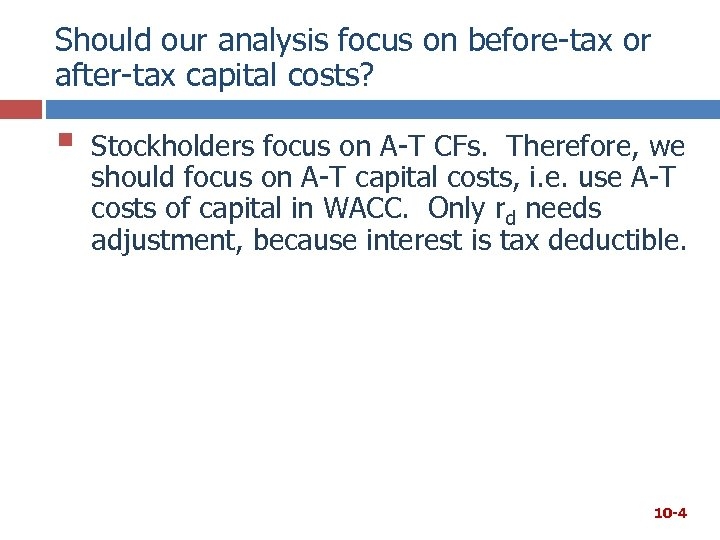Should our analysis focus on before-tax or after-tax capital costs? § Stockholders focus on A-T CFs. Therefore, we should focus on A-T capital costs, i. e. use A-T costs of capital in WACC. Only rd needs adjustment, because interest is tax deductible. 10 -4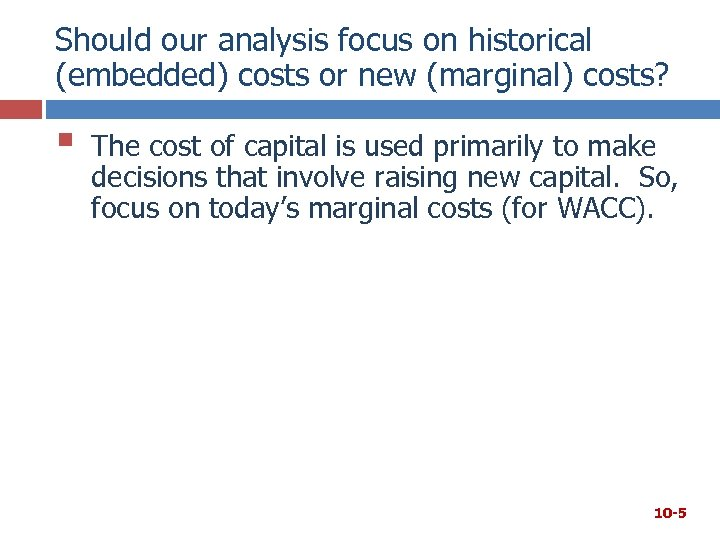Should our analysis focus on historical (embedded) costs or new (marginal) costs? § The cost of capital is used primarily to make decisions that involve raising new capital. So, focus on today’s marginal costs (for WACC). 10 -5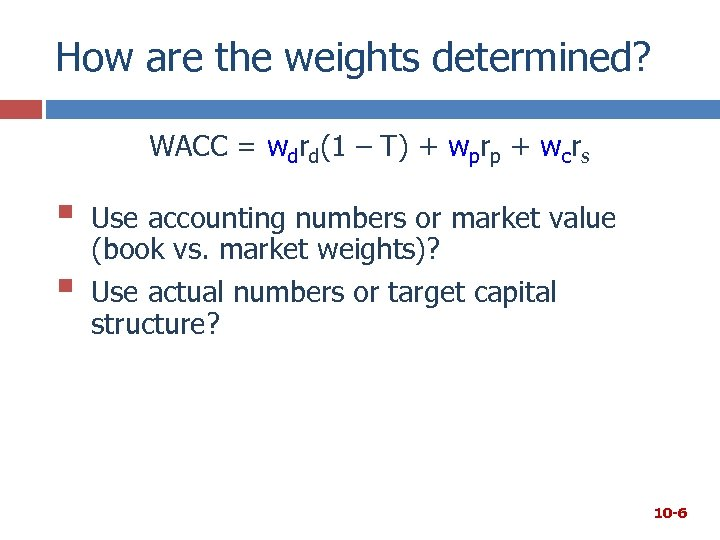How are the weights determined? WACC = wdrd(1 – T) + wprp + wcrs § § Use accounting numbers or market value (book vs. market weights)? Use actual numbers or target capital structure? 10 -6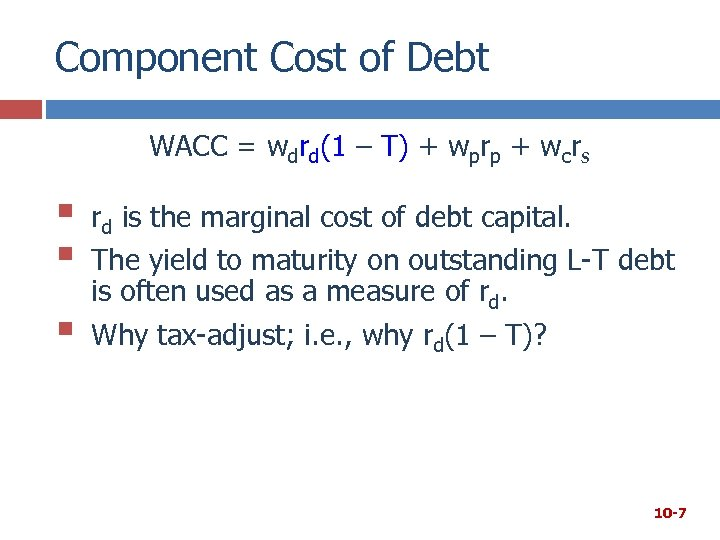Component Cost of Debt WACC = wdrd(1 – T) + wprp + wcrs § § § rd is the marginal cost of debt capital. The yield to maturity on outstanding L-T debt is often used as a measure of rd. Why tax-adjust; i. e. , why rd(1 – T)? 10 -7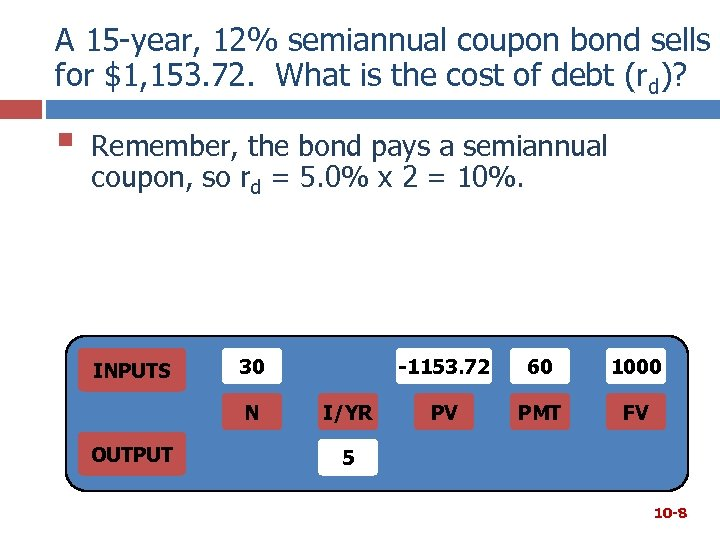A 15 -year, 12% semiannual coupon bond sells for \$1, 153. 72. What is the cost of debt (rd)? § Remember, the bond pays a semiannual coupon, so rd = 5. 0% x 2 = 10%. INPUTS 30 N OUTPUT -1153. 72 I/YR 60 1000 PV PMT FV 5 10 -8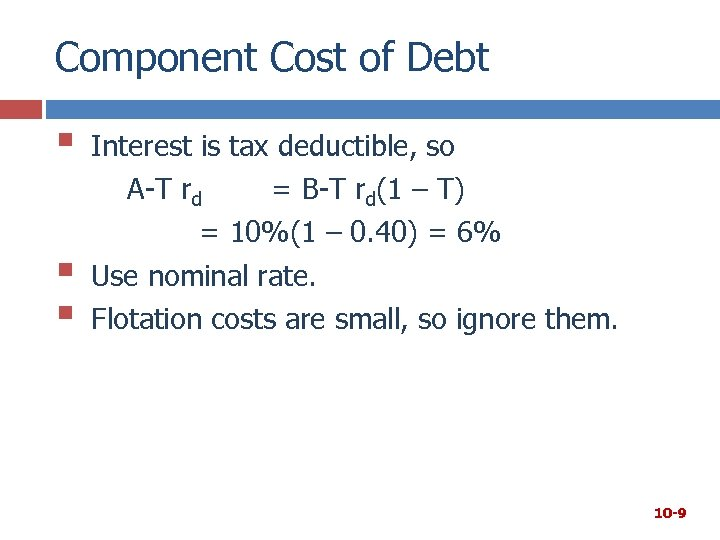Component Cost of Debt § § § Interest is tax deductible, so A-T rd = B-T rd(1 – T) = 10%(1 – 0. 40) = 6% Use nominal rate. Flotation costs are small, so ignore them. 10 -9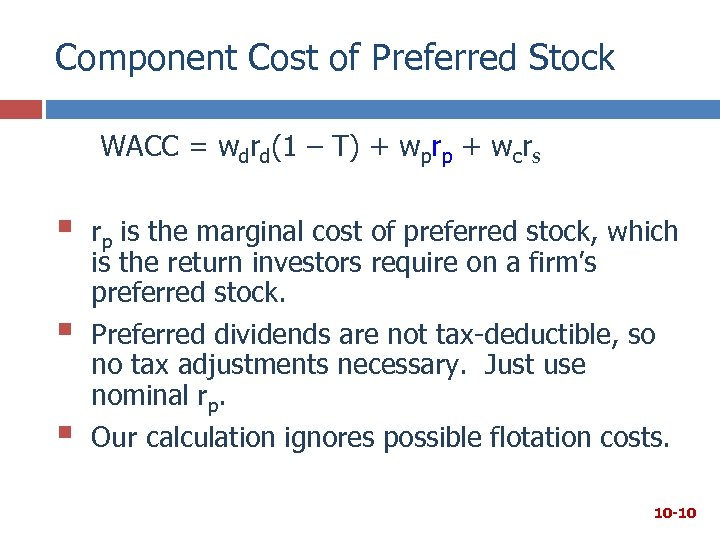Component Cost of Preferred Stock WACC = wdrd(1 – T) + wprp + wcrs § § § rp is the marginal cost of preferred stock, which is the return investors require on a firm’s preferred stock. Preferred dividends are not tax-deductible, so no tax adjustments necessary. Just use nominal rp. Our calculation ignores possible flotation costs. 10 -10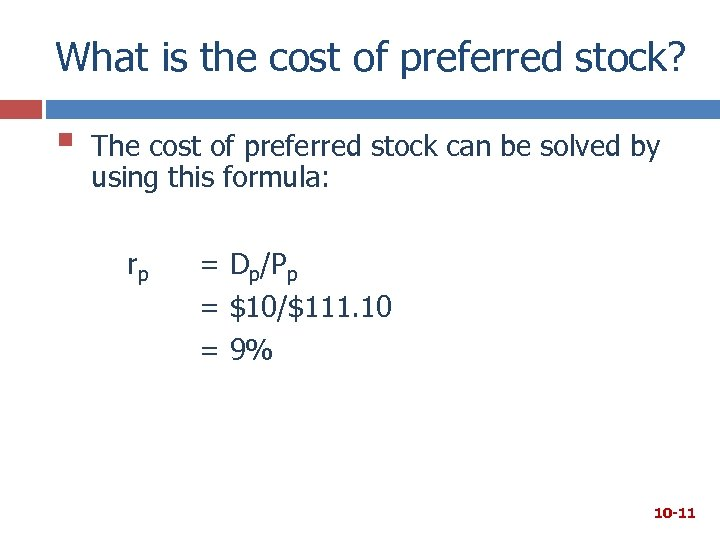What is the cost of preferred stock? § The cost of preferred stock can be solved by using this formula: rp = Dp/Pp = \$10/\$111. 10 = 9% 10 -11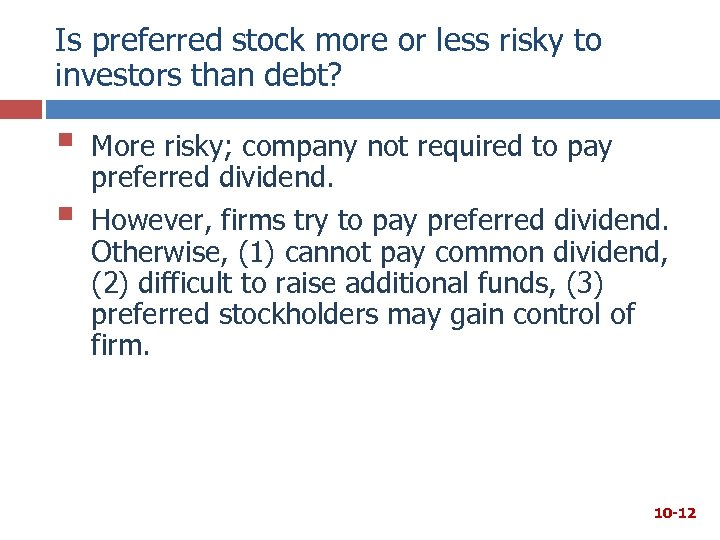Is preferred stock more or less risky to investors than debt? § § More risky; company not required to pay preferred dividend. However, firms try to pay preferred dividend. Otherwise, (1) cannot pay common dividend, (2) difficult to raise additional funds, (3) preferred stockholders may gain control of firm. 10 -12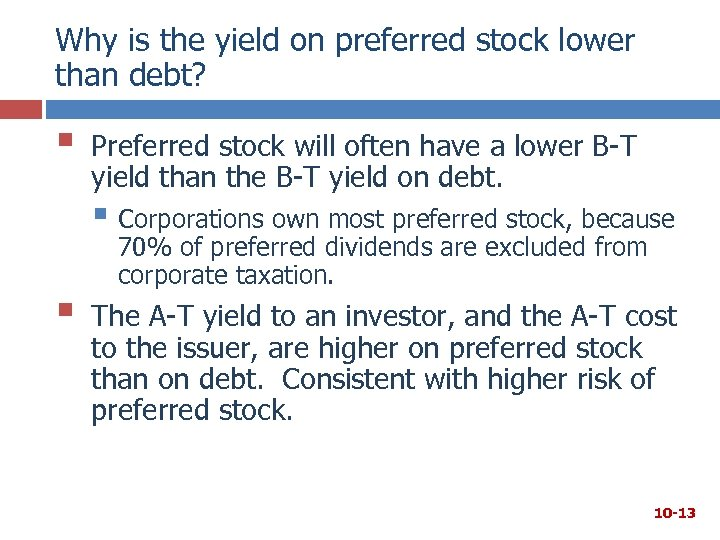Why is the yield on preferred stock lower than debt? § Preferred stock will often have a lower B-T yield than the B-T yield on debt. § Corporations own most preferred stock, because § 70% of preferred dividends are excluded from corporate taxation. The A-T yield to an investor, and the A-T cost to the issuer, are higher on preferred stock than on debt. Consistent with higher risk of preferred stock. 10 -13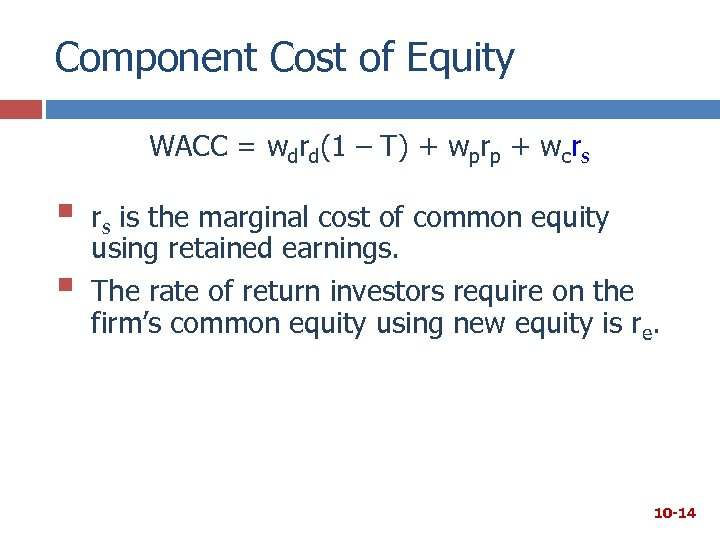Component Cost of Equity WACC = wdrd(1 – T) + wprp + wcrs § § rs is the marginal cost of common equity using retained earnings. The rate of return investors require on the firm’s common equity using new equity is re. 10 -14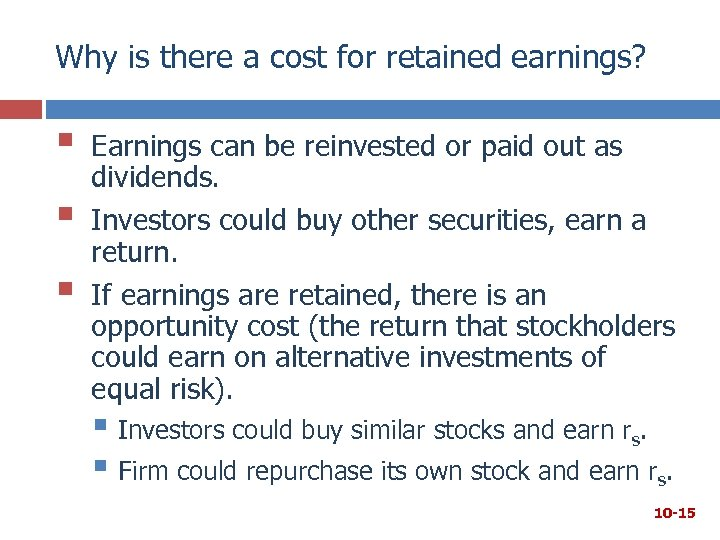Why is there a cost for retained earnings? § § § Earnings can be reinvested or paid out as dividends. Investors could buy other securities, earn a return. If earnings are retained, there is an opportunity cost (the return that stockholders could earn on alternative investments of equal risk). § Investors could buy similar stocks and earn rs. § Firm could repurchase its own stock and earn rs. 10 -15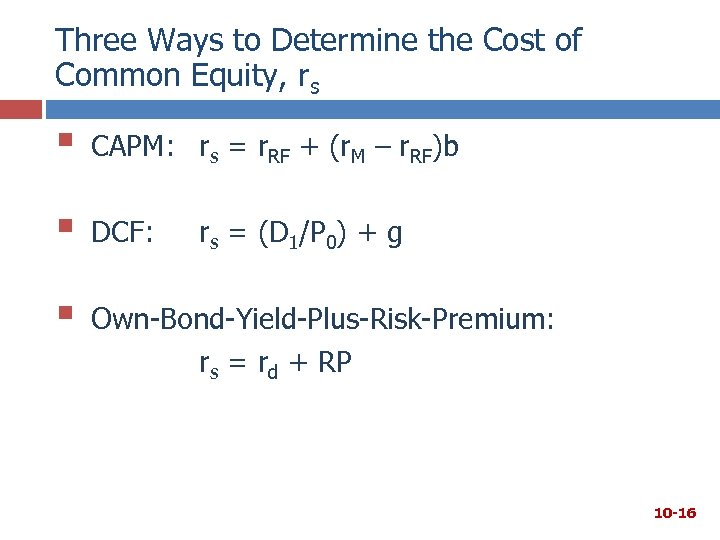Three Ways to Determine the Cost of Common Equity, rs § CAPM: rs = r. RF + (r. M – r. RF)b § DCF: § Own-Bond-Yield-Plus-Risk-Premium: rs = rd + RP rs = (D 1/P 0) + g 10 -16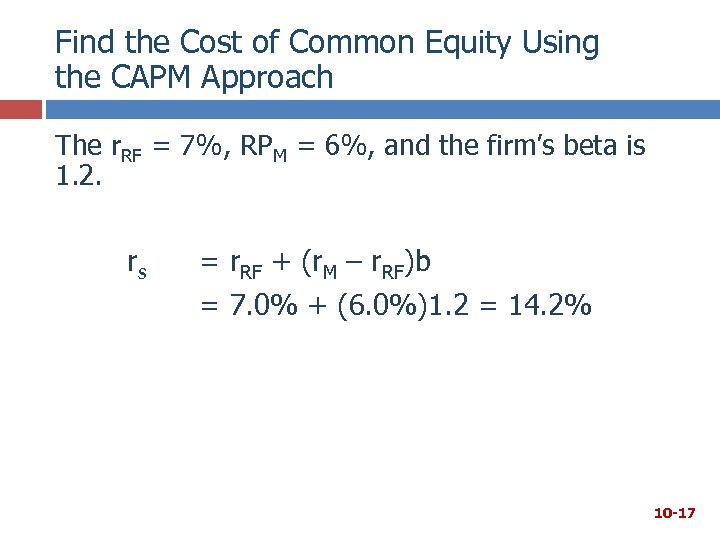Find the Cost of Common Equity Using the CAPM Approach The r. RF = 7%, RPM = 6%, and the firm’s beta is 1. 2. rs = r. RF + (r. M – r. RF)b = 7. 0% + (6. 0%)1. 2 = 14. 2% 10 -17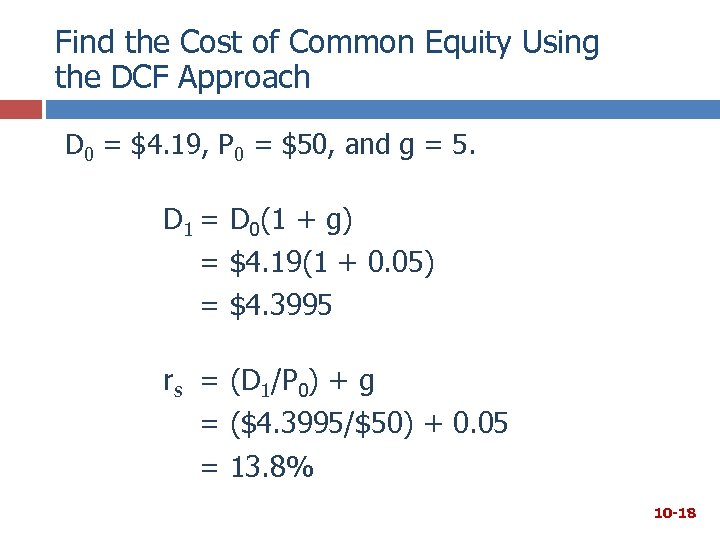Find the Cost of Common Equity Using the DCF Approach D 0 = \$4. 19, P 0 = \$50, and g = 5. D 1 = D 0(1 + g) = \$4. 19(1 + 0. 05) = \$4. 3995 rs = (D 1/P 0) + g = (\$4. 3995/\$50) + 0. 05 = 13. 8% 10 -18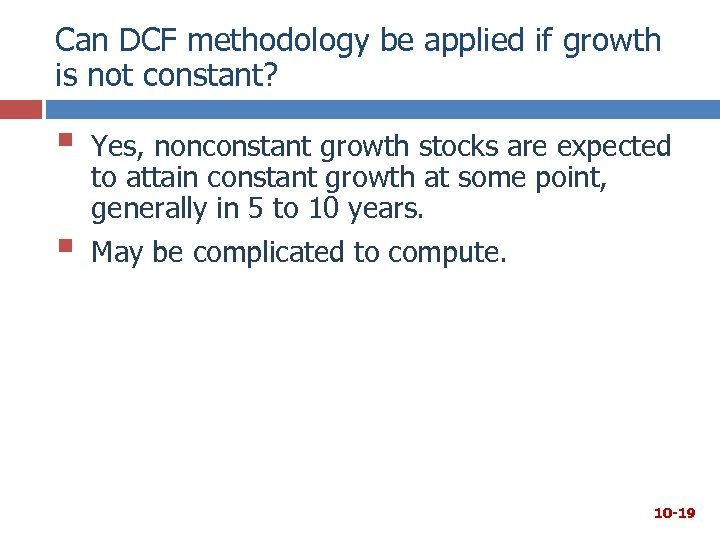Can DCF methodology be applied if growth is not constant? § § Yes, nonconstant growth stocks are expected to attain constant growth at some point, generally in 5 to 10 years. May be complicated to compute. 10 -19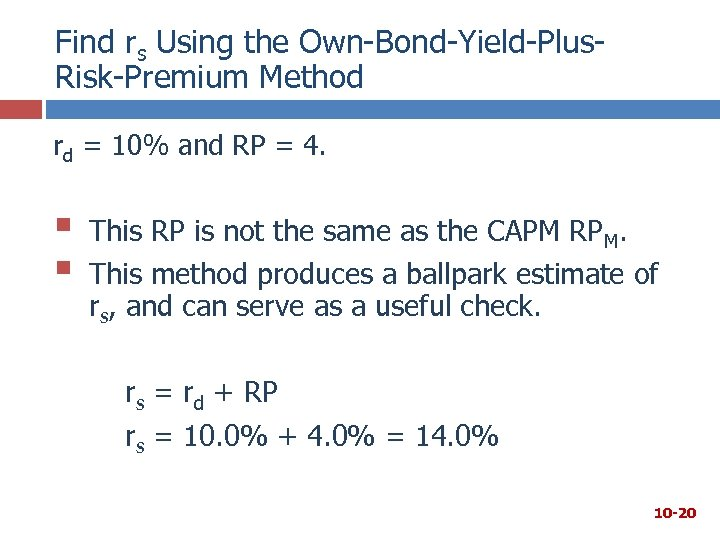Find rs Using the Own-Bond-Yield-Plus. Risk-Premium Method rd = 10% and RP = 4. § § This RP is not the same as the CAPM RPM. This method produces a ballpark estimate of rs, and can serve as a useful check. rs = rd + RP rs = 10. 0% + 4. 0% = 14. 0% 10 -20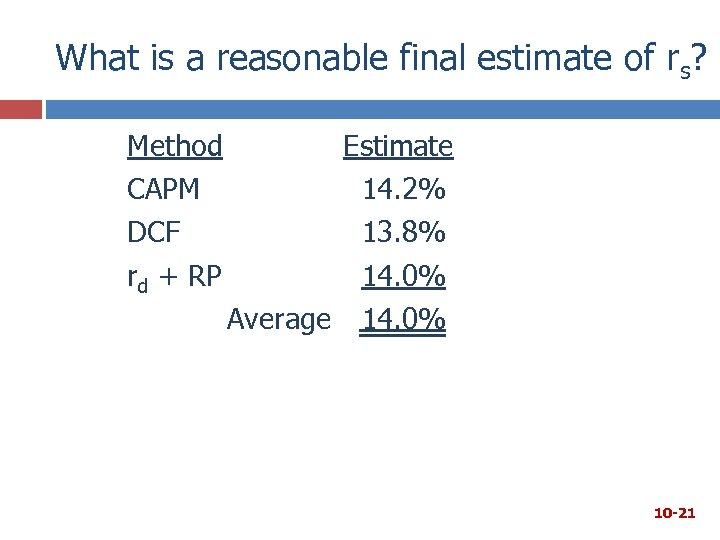What is a reasonable final estimate of rs? Method CAPM DCF rd + RP Estimate 14. 2% 13. 8% 14. 0% Average 14. 0% 10 -21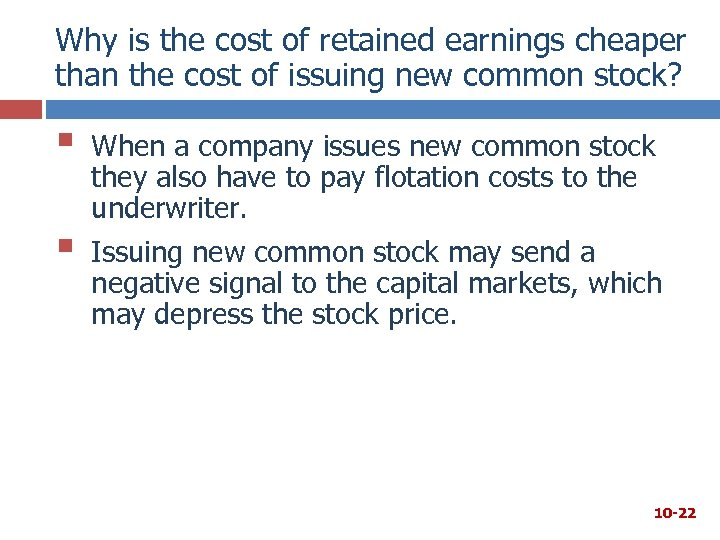Why is the cost of retained earnings cheaper than the cost of issuing new common stock? § § When a company issues new common stock they also have to pay flotation costs to the underwriter. Issuing new common stock may send a negative signal to the capital markets, which may depress the stock price. 10 -22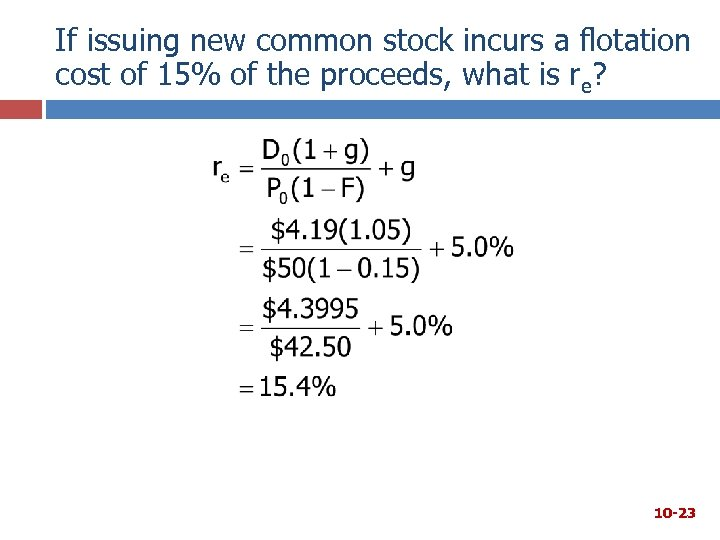If issuing new common stock incurs a flotation cost of 15% of the proceeds, what is re? 10 -23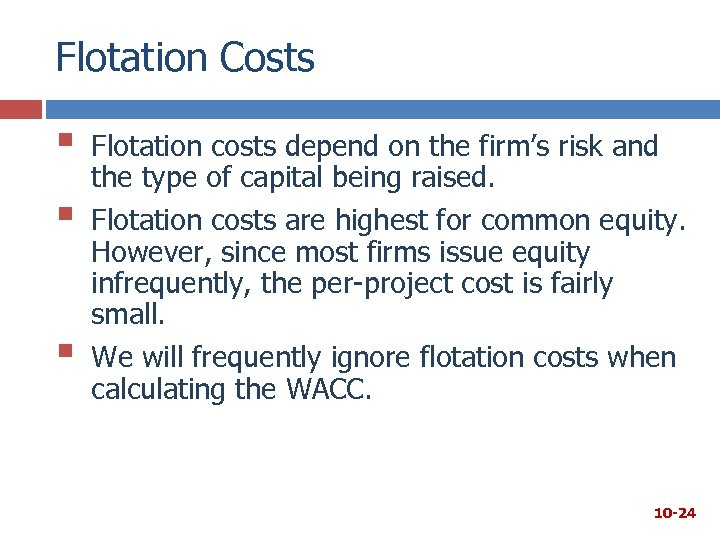Flotation Costs § § § Flotation costs depend on the firm’s risk and the type of capital being raised. Flotation costs are highest for common equity. However, since most firms issue equity infrequently, the per-project cost is fairly small. We will frequently ignore flotation costs when calculating the WACC. 10 -24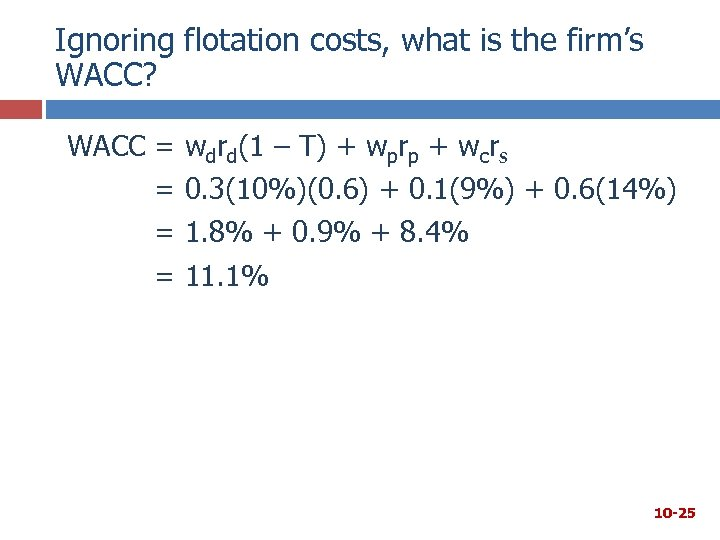Ignoring flotation costs, what is the firm’s WACC? WACC = = wdrd(1 – T) + wprp + wcrs 0. 3(10%)(0. 6) + 0. 1(9%) + 0. 6(14%) 1. 8% + 0. 9% + 8. 4% 11. 1% 10 -25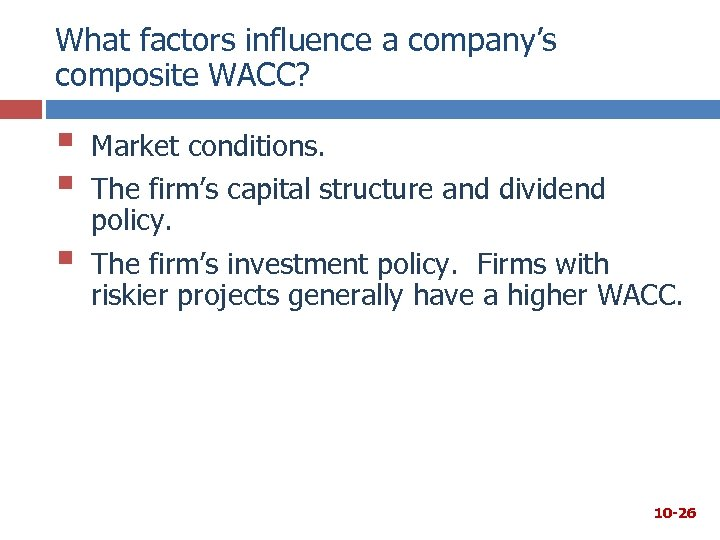What factors influence a company’s composite WACC? § § § Market conditions. The firm’s capital structure and dividend policy. The firm’s investment policy. Firms with riskier projects generally have a higher WACC. 10 -26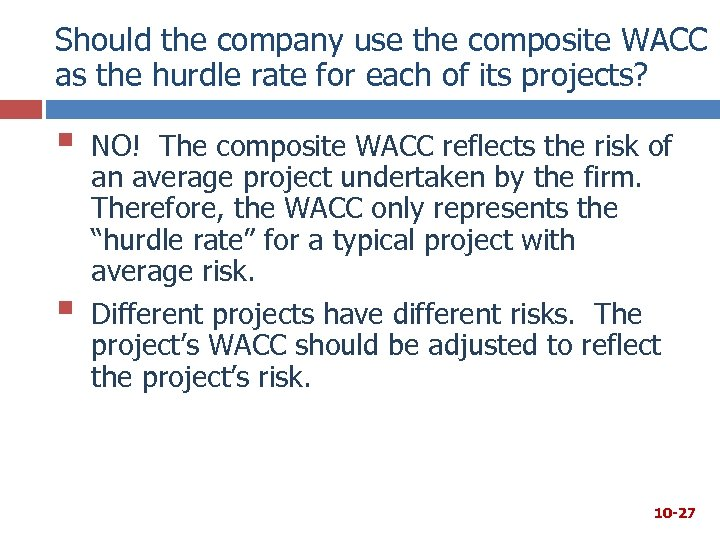Should the company use the composite WACC as the hurdle rate for each of its projects? § § NO! The composite WACC reflects the risk of an average project undertaken by the firm. Therefore, the WACC only represents the “hurdle rate” for a typical project with average risk. Different projects have different risks. The project’s WACC should be adjusted to reflect the project’s risk. 10 -27